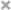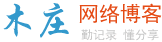# HTML5 新表单元素

HTML5 <datalist>元素

<datalist>元素规定输入域的选项列表

<datalist>属性规定form或input域应该拥有自动完成功能。当用户在自动完成域中开始输入时，浏览器应该在该域中显示填写的选项；

 1234567891011121314151617181920212223242526 ```<``html``>``    ``<``head``>``        ``<``meta` `charset``=``"utf-8"` `/>``        ``<``title``>自选教程(如约智惠.com)``        ` `    ````    ``<``body` `> ``        ``<``form` `action``=``"demo-form.php"` `method``=``"get"``>``            ``<``input` `list``=``"browsers"` `name``=``"browser"``>``            ``<``datalist` `id``=``"browsers"``>``                ``<``option` `value``=``"Internet Explorer"``>``                ``<``option` `value``=``"Firefox"``>``                ``<``option` `value``=``"Chrome"``>``                ``<``option` `value``=``"Opera"``>``                ``<``option` `value``=``"Safari"``>``            ````            ``<``input` `type``=``"submit"``>``        ````        ` `        ``<``p``>``            ``<``strong``>注意：Internet Explorer 9， Safari 不支持 datalist 标签。``        ````        ` `    `````

HTML5 <keygen>元素

<keygen> 元素的作用是提供一种验证用户的可靠方法。

<keygen>标签规定用于表单的密钥对生成器字段。

 123456789101112131415161718192021 ```<``html``>``    ``<``head``>``        ``<``meta` `charset``=``"utf-8"` `/>``        ``<``title``>自选教程(如约智惠.com)``        ` `    ````    ``<``body` `> ``        ``<``form` `action``=``"demo-form.php"` `method``=``"get"``>``            ``用户名：<``input` `list``=``"text"` `name``=``"usr_name"``>``            ``密码：<``keygen` `name``=``"security"``>``            ````            ``<``input` `type``=``"submit"``>``        ````        ` `        ``<``p``>``            ``<``strong``>注意：Internet Explorer 不支持 keygen标签。``        ````        ` `    `````

HTML5 <output> 元素

<output>元素用于不同类型的输出，比如计算或脚本输出：

 1234567891011121314151617181920 ```<``html``>``    ``<``head``>``        ``<``meta` `charset``=``"utf-8"` `/>``        ``<``title``>自选教程(如约智惠.com)``        ` `    ````    ``<``body` `> ``        ``<``form` `oninput``=``"x.value=parseInt(a.value)+parseInt(b.value)"``> 0``            ``<``input` `type``=``"range"` `id``=``"a"` `value``=``"50"``> 100``            ``+<``input` `type``=``"number"` `id``=``"b"` `value``=``"50"``>``            ``=<``output` `name``=``"x"` `for``=``"a b"``>``        ````        ` `        ``<``p``>``            ``<``strong``>注意：Internet Explorer 不支持 output 标签。``        ````        ` `    `````

## HTML5 新表单元素

<datalist><input>标签定义选项列表。请与 input 元素配合使用该元素，来定义 input 可能的值。
<keygen><keygen> 标签规定用于表单的密钥对生成器字段。
<output><output> 标签定义不同类型的输出，比如脚本的输出。

web sql数据库的使用教程

HTML5利用canvas绘制哆啦a梦头部（代码实例）

HTML5 touch事件实现触屏页面上下滑动

h5怎么实现在线预览pdf

h5 canvas实现圆形动态加载进度实例

css3如何设置placeholder的样式

HTML5游戏框架cngamejs开发实录-碰撞检测模块篇

HTML5中localstorage本地存储的示例### 评论

• 欢迎访问木庄网络博客
• 可复制：代码框内的文字。
• 方法：Ctrl+C。